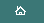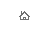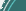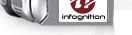blog tags: directshow (8) programming (11) super_resolution (6) video (2) video_enhancer (12) all (40) About: I'm Dmitry Popov, lead developer and director of Infognition. Known in the interwebs as Dee Mon since 1997. You could see me as thedeemon on reddit or LiveJournal.Articles Technology Blog News Company
Blog
 Dependent types in half of D July 30, 2015 Recently I saw a talk by one of active Idris contributors: David Christiansen - Coding for Types: The Universe Pattern in Idris where he shows some uses of dependent types. I'd like to use some of those examples to demonstrate that we actually have dependent types in D, or, really, in part of it. First let me show some original examples in Idris, a full blown depentently typed language, and then we'll translate them to D closely. In the talk David says that in dependently typed languages we have types as first class values: you can create them on the fly, return from functions, store in data structures and pass around, however you shouldn't pattern-match on them because if we allow pattern-matching on types we lose free theorems (id may start to return 42 for all natural arguments) and this prevents proper type erasure when compiling the programs. I'm not sure if matching on types is actually banned in Idris now, I remember a few versions ago it actually worked, at least in simple cases. But sometimes doing different things depending on type passed is what we do want, and for this cases David describes what he calls The Universe Pattern: you take the types you wish to match on, encode them in a simple data type (as in AST), match on this data type, and when you need actual types use a mapping function from this encoding to actual types. For example, let's say we work with relational tables where each value can be either some text, an integer or a real number. We encode this with an algebraic data type: ```data Column = TEXT | REAL | INTEGER ``` and immediately define a mapping from its values to actual types: ```interpCol : Column -> Type interpCol TEXT -> String interpCol REAL -> Double interpCol INTEGER -> Integer ``` Now, if we want to convert a value of one of those types to a string we can use a function like this: ```printCol : (c : Column) -> interpCol c -> String printCol TEXT x = x printCol REAL x = doubleToString x printCol INTEGER x = intToString x ``` This is a function with two arguments (returning a String). First argument `c` has the type `Column`, and type of the second argument depends on value of the first argument, it is calculated by applying function `interpCol` to `c` (this function returns a type, so `interpCol c` is a type). This is a classical dependent type. Now you can call `printCol TEXT "hi"` or `printCol INTEGER 77` but you can't do `printCol TEXT 77`, this would be a type error caught by the compiler. Similarly David describes how to define a table schema and map it to an actual data type of one table row. ```Table : Type Table = List (String, Column) interp : Table -> Type interp tbl = HList (map (interpCol . snd) tbl) ``` For instance, this is how a particular table and its schema are defined as data: ```PeopleSchema : Table PeopleSchema = [("Name", TEXT), ("Hamsters", INTEGER)] People : List (interp PeopleSchema) People = [["David", 1], ["Edwin", 0]] ``` In this schema there are two columns. Calling `interp PeopleSchema` returns `HList [String, Integer]`, this is a type of a heterogeneous list with exactly two elements where the first one is a string and the second is an integer. Each list of this type contains a row of our table. Instead of `HList` there could be a simple tuple but David just reused `HList` he defined a few minutes earlier in the talk. Now that we can map a table schema definition to a data type for its rows we can calculate total number of hamsters in this table and define a function for converting arbitrary table rows to strings. ```countHamsters : List (interp PeopleSchema) -> Integer countHamsters [] = 0 countHamsters ([_, h] :: xs) = h + countHamsters xs printRow : (tbl : Table) -> interp tbl -> String printRow [] [] = "" printRow ((_, t) :: vs) (x :: xs) = printCol t x ++ "\t" ++ printRow vx xs ``` Here `countHamsters` works with tables of concrete schema `PeopleSchema`, while `printRow` can work with any table: it takes schema definition value `tbl` and one row of data, whose type is defined by evaluating `interp tbl`. This is another good example of a dependent type. Both of those arguments are lists (one ordinary and one heterogeneous), for each column `printRow` takes a `Column` value from the schema definition (it encodes the type of actual value) and the actual value from the data row. It passes them to `printCol` that converts the value to a string properly. The rest is just trivial mechanics of looping recursively over the lists and combining the results in one tab-separated string. Well, dependent types in Idris are not surprising, of course, being the key feature of the language. But did you know dependent types already got to mainstream being available in C++ and its younger brother D? Just not in whole language, only in half of it. We're used to thinking that D is a compiled language but it's also an interpreted language where the interpreter is built into the compiler and runs during the compilation stage, allowing CTFE (compile-time function execution) and many powerful metaprogramming features. Modern C++ also followed D in this direction. And at this interpreted stage we have real dependent types. Let's translate David's examples to D closely, to demonstrate the correspondence. First, we had a data type `Column` encoding types of table cells, and its mapping to actual types. ```enum Column { TEXT, REAL, INTEGER } template interpCol(Column col) { // Column -> Type static if (col==Column.INTEGER) alias interpCol = int; else static if (col==Column.REAL) alias interpCol = double; else static if (col==Column.TEXT) alias interpCol = string; } ``` Then we had function `printCol` with a dependent type `(c : Column) -> interpCol c -> String`. ```string printCol(Column c, alias x)() if (is(typeof(x) == interpCol!(c))) { static if (c==Column.TEXT) return x; else return x.to!string; } ``` This is a real function with two arguments, returning a string. As in the original, the first argument `c` has the type `Column` and the type of the second argument can vary, it depends on value `c` and must be equal to result of evaluation of `interpCol!(c)`, the check for this is written explicitly and performed statically, trying to pass a value of a wrong type will cause a type error. For example, we can call `printCol!(Column.INTEGER, 55)` and `printCol!(Column.TEXT, "hi")` just fine but we cannot call `printCol!(Column.TEXT, 55)`, this does not compile. The secret sauce here is two sets of parentheses after `printCol`: the arguments are listed inside the first pair of parentheses, this is where compile-time arguments are passed. The `static if` also does its job at compile time. Now let's learn how to define table schemas, corresponding data and functions working with such tables. For compile-time lists there is a native D type `TypeTuple` (also known as AliasSeq starting from next compiler release), originally it appeared to define lists of compile-time arguments to templates, so it's heterogeneous and its elements can be types, numbers, strings, struct instances, other templates... virtually anything. The standard library contains functions for working with such lists - filter, map, reverse, etc. We're going to need map, it's called `staticMap` there. `TypeTuple` has one peculiarity: it flattens automatically, so a list of lists becomes just a single long list of all the elements. That's not what we want, so we'll make a wrapper: ```struct List(Things...) { // compile-time HList alias contents = Things; } alias getType(x...) = typeof(x); alias listValueTypes(lst) = List!(staticMap!(getType, lst.contents)); ``` A compile-time function `listValueTypes` will turn a heterogeneous list of values into list of their types, we'll need it to type check those. In the original Idris code one table column was described in the schema by a tuple `(String, Column)` and the `Column` value was extracted with `snd`. Instead, we'll define a special structure NC (named column) where contents can be addressed by names. ```struct NC(string A, Column B) { // named column alias name = A; alias col = B; } alias columnType(nc) = interpCol!(nc.col); alias interp(tbl) = List!(staticMap!(columnType, tbl.contents)); ``` As a `Table` (schema definition data type) we'll use `List` containing arbitraty number of such named columns. `columnType` will help us map a column definition to an actual type, and `interp` will map list of column definitions into a list of actual data types, just like in the Idris original. Here's how converting arbitrary table row to a string will look like: ```string printRow(tbl, xs)() if (is(listValueTypes!xs == interp!tbl)) { string[tbl.contents.length] res; foreach(i, x; xs.contents) res[i] = printCol!(tbl.contents[i].col, x); return res[].join("\t"); } ``` just like in Idris source, this function has two arguments: the schema `tbl` and a row of data having a type that's calculated by applying `interp` to `tbl`. Type checking here is performed explicitly by evaluating `listValueTypes!xs` and `interp!tbl` and comparing them. Inside the function we loop over the lists of columns and call `printCol` passing the column type encoding (a Column value from the schema) and actual data value from the data row. Note that on different iterations of the loop the type of `x` will be different. Definition of a particular table with hamsters and counting them will look like this: ```alias PeopleSchema = List!( NC!("Name", Column.TEXT), NC!("Hamsters", Column.INTEGER) ); int countHamsters(rows...)() { int sum = 0; foreach(r; rows) { static assert(is(listValueTypes!r == interp!PeopleSchema)); sum += r.contents; } return sum; } ``` The `countHamsters` function takes a list with data rows. Here I used the built-in heterogeneous compile-time list (TypeTuple), and since each element of it can have its own type we check the types inside the loop. For each data row `r` we calculate the list of its element types and compare with result of mapping our schema to actual types, it's the same what Idris compiler did. Let's run it on concrete data: ```void main(string[] argv) { alias People = TypeTuple!( List!("David", 10), List!("Edwin", 11) ); int n = countHamsters!People; string s = printRow!(PeopleSchema, People); writeln("hamsters: ", n, ", first row: ", s); } ``` It compiles and when run outputs `hamsters: 21, first row: David 10` Full source code, with compilation and output, can be found here. In both Idris and D code above we had all the data in the source code but we didn't have to do it this way, we can define schema and data in a separate data file. In Idris we can use input-output to read the schema and data before processing. This is a fully dependently typed language where types can depend on values calculated in run-time. In D we can read the input and parse it at compile-time, there are ready means to do that (see Pegged library). All the interesting dependently-typed stuff happens at compile-time in D, but since this is where its interpreter is working, "compile-time" for ordinary D is "run-time" for the interpreted meta-programming part of D, and the types can depend on values there. We can use it as we would use Python or Ruby: just write "rdmd program.d arguments" and it will compile and run it in one go, so both stages (compile-time and run-time, interpeted D and compiled D) are executed together, and at least at one of the stages we have dependent types. Just like Idris, it may be not too practical but surely fun!Comments (24) tags: programming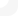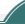Copyright © 2001-2009 Dee Mon, 2009-2020 Infognition Co. Ltd.